# basics of thermodynamics- chemical engg.

3. May 2017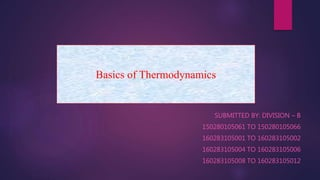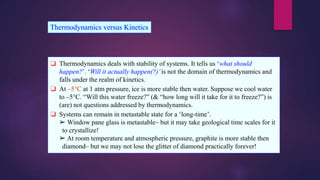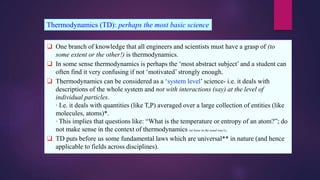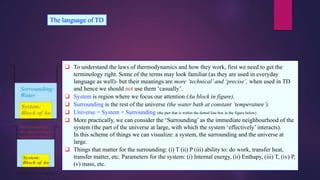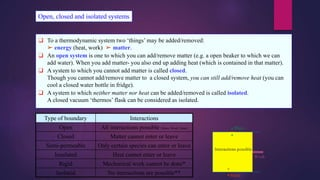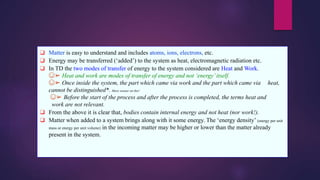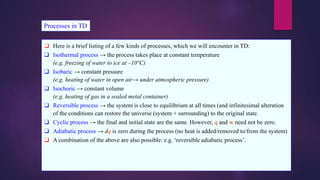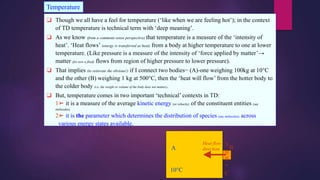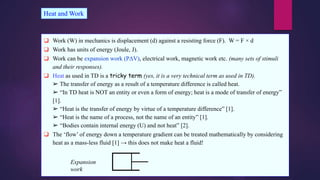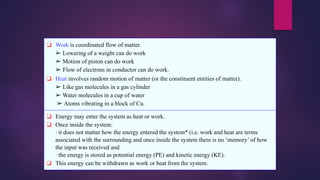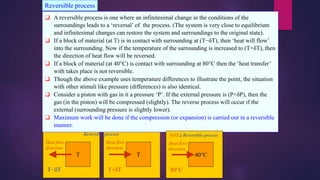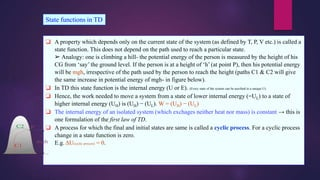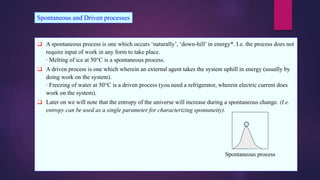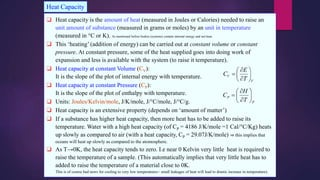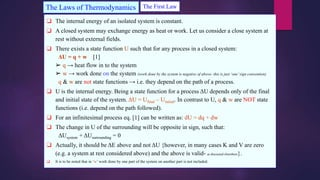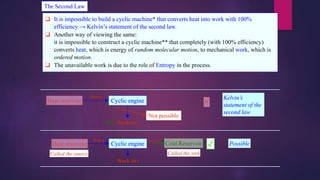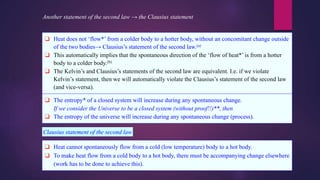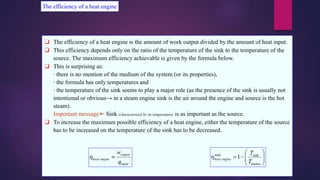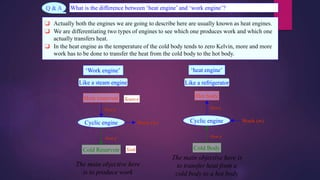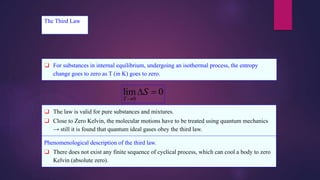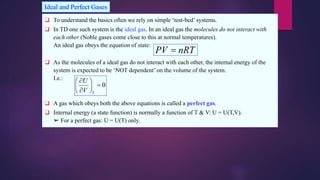1 von 22

### basics of thermodynamics- chemical engg.

• 1. SUBMITTED BY: DIVISION – B 150280105061 TO 150280105066 160283105001 TO 160283105002 160283105004 TO 160283105006 160283105008 TO 160283105012 Basics of Thermodynamics
• 2. ❑ Thermodynamics deals with stability of systems. It tells us ‘what should happen?’. ‘Will it actually happen(?)’is not the domain of thermodynamics and falls under the realm of kinetics. ❑ At –5°C at 1 atm pressure, ice is more stable then water. Suppose we cool water to –5°C. “Will this water freeze?” (& “how long will it take for it to freeze?”) is (are) not questions addressed by thermodynamics. ❑ Systems can remain in metastable state for a ‘long-time’. ➢ Window pane glass is metastable– but it may take geological time scales for it to crystallize! ➢ At room temperature and atmospheric pressure, graphite is more stable then diamond– but we may not lose the glitter of diamond practically forever! Thermodynamics versus Kinetics
• 3. ❑ One branch of knowledge that all engineers and scientists must have a grasp of (to some extent or the other!) is thermodynamics. ❑ In some sense thermodynamics is perhaps the ‘most abstract subject’ and a student can often find it very confusing if not ‘motivated’ strongly enough. ❑ Thermodynamics can be considered as a ‘system level’ science- i.e. it deals with descriptions of the whole system and not with interactions (say) at the level of individual particles. ∙ I.e. it deals with quantities (like T,P) averaged over a large collection of entities (like molecules, atoms)*. ∙ This implies that questions like: “What is the temperature or entropy of an atom?”; do not make sense in the context of thermodynamics (at lease in the usual way!). ❑ TD puts before us some fundamental laws which are universal** in nature (and hence applicable to fields across disciplines). Thermodynamics (TD): perhaps the most basic science
• 4. ❑ To understand the laws of thermodynamics and how they work, first we need to get the terminology right. Some of the terms may look familiar (as they are used in everyday language as well)- but their meanings are more ‘technical’and ‘precise’, when used in TD and hence we should not use them ‘casually’. ❑ System is region where we focus our attention (Au block in figure). ❑ Surrounding is the rest of the universe (the water bath at constant ‘temperature’). ❑ Universe = System + Surrounding (the part that is within the dotted line box in the figure below) ❑ More practically, we can consider the ‘Surrounding’ as the immediate neighbourhood of the system (the part of the universe at large, with which the system ‘effectively’ interacts). In this scheme of things we can visualize: a system, the surrounding and the universe at large. ❑ Things that matter for the surrounding: (i) T (ii) P (iii) ability to: do work, transfer heat, transfer matter, etc. Parameters for the system: (i) Internal energy, (ii) Enthapy, (iii) T, (iv) P, (v) mass, etc. The language of TD
• 5. ❑ To a thermodynamic system two ‘things’ may be added/removed: ➢ energy (heat, work) ➢ matter. ❑ An open system is one to which you can add/remove matter (e.g. a open beaker to which we can add water). When you add matter- you also end up adding heat (which is contained in that matter). ❑ A system to which you cannot add matter is called closed. Though you cannot add/remove matter to a closed system, you can still add/remove heat (you can cool a closed water bottle in fridge). ❑ A system to which neither matter nor heat can be added/removed is called isolated. A closed vacuum ‘thermos’ flask can be considered as isolated. Open, closed and isolated systems Type of boundary Interactions Open All interactions possible (Mass, Work, Heat) Closed Matter cannot enter or leave Semi-permeable Only certain species can enter or leave Insulated Heat cannot enter or leave Rigid Mechanical work cannot be done* Isolated No interactions are possible**
• 6. ❑ Matter is easy to understand and includes atoms, ions, electrons, etc. ❑ Energy may be transferred (‘added’) to the system as heat, electromagnetic radiation etc. ❑ In TD the two modes of transfer of energy to the system considered are Heat and Work. ☺➢ Heat and work are modes of transfer of energy and not ‘energy’itself. ☺➢ Once inside the system, the part which came via work and the part which came via heat, cannot be distinguished*. More sooner on this! ☺➢ Before the start of the process and after the process is completed, the terms heat and work are not relevant. ❑ From the above it is clear that, bodies contain internal energy and not heat (nor work!). ❑ Matter when added to a system brings along with it some energy. The ‘energy density’ (energy per unit mass or energy per unit volume) in the incoming matter may be higher or lower than the matter already present in the system.
• 7. ❑ Here is a brief listing of a few kinds of processes, which we will encounter in TD: ❑ Isothermal process → the process takes place at constant temperature (e.g. freezing of water to ice at –10°C) ❑ Isobaric → constant pressure (e.g. heating of water in open air→ under atmospheric pressure) ❑ Isochoric → constant volume (e.g. heating of gas in a sealed metal container) ❑ Reversible process → the system is close to equilibrium at all times (and infinitesimal alteration of the conditions can restore the universe (system + surrounding) to the original state. ❑ Cyclic process → the final and initial state are the same. However, q and w need not be zero. ❑ Adiabatic process → dq is zero during the process (no heat is added/removed to/from the system) ❑ A combination of the above are also possible: e.g. ‘reversible adiabatic process’. Processes in TD
• 8. ❑ Though we all have a feel for temperature (‘like when we are feeling hot’); in the context of TD temperature is technical term with ‘deep meaning’. ❑ As we know (from a commons sense perspective) that temperature is a measure of the ‘intensity of heat’. ‘Heat flows’ (energy is transferred as heat) from a body at higher temperature to one at lower temperature. (Like pressure is a measure of the intensity of ‘force applied by matter’→ matter (for now a fluid) flows from region of higher pressure to lower pressure). ❑ That implies (to reiterate the obvious!) if I connect two bodies− (A)-one weighing 100kg at 10°C and the other (B) weighing 1 kg at 500°C, then the ‘heat will flow’ from the hotter body to the colder body (i.e. the weight or volume of the body does not matter). ❑ But, temperature comes in two important ‘technical’ contexts in TD: 1➢ it is a measure of the average kinetic energy (or velocity) of the constituent entities (say molecules) 2➢ it is the parameter which determines the distribution of species (say molecules) across various energy states available. Temperature 5 0 0 ° C Heat flow direction 10°C A B
• 9. ❑ Pressure* is force per unit area (usually exerted by a fluid on a wall**). ❑ It is the momentum transferred (say on a flat wall by molecules of a gas) per unit area, per unit time. (In the case of gas molecules it is the average momentum transferred per unit area per unit time on to the flat wall). ➢ P = momentum transferred/area/time. ❑ Pressure is related to momentum, while temperature is related to kinetic energy. Pressure Wallofacontainer ‘Crude schematic’ of particles impinging on a wall.
• 10. ❑ Work (W) in mechanics is displacement (d) against a resisting force (F). W = F × d ❑ Work has units of energy (Joule, J). ❑ Work can be expansion work (PΔV), electrical work, magnetic work etc. (many sets of stimuli and their responses). ❑ Heat as used in TD is a tricky term (yes, it is a very technical term as used in TD). ➢ The transfer of energy as a result of a temperature difference is called heat. ➢ “In TD heat is NOT an entity or even a form of energy; heat is a mode of transfer of energy” . ➢ “Heat is the transfer of energy by virtue of a temperature difference” . ➢ “Heat is the name of a process, not the name of an entity” . ➢ “Bodies contain internal energy (U) and not heat” . ❑ The ‘flow’ of energy down a temperature gradient can be treated mathematically by considering heat as a mass-less fluid  → this does not make heat a fluid! Heat and Work Expansion work
• 11. ❑ Work is coordinated flow of matter. ➢ Lowering of a weight can do work ➢ Motion of piston can do work ➢ Flow of electrons in conductor can do work. ❑ Heat involves random motion of matter (or the constituent entities of matter). ➢ Like gas molecules in a gas cylinder ➢ Water molecules in a cup of water ➢ Atoms vibrating in a block of Cu. ❑ Energy may enter the system as heat or work. ❑ Once inside the system: ∙ it does not matter how the energy entered the system* (i.e. work and heat are terms associated with the surrounding and once inside the system there is no ‘memory’ of how the input was received and ∙ the energy is stored as potential energy (PE) and kinetic energy (KE). ❑ This energy can be withdrawn as work or heat from the system.
• 12. ❑ A reversible process is one where an infinitesimal change in the conditions of the surroundings leads to a ‘reversal’ of the process. (The system is very close to equilibrium and infinitesimal changes can restore the system and surroundings to the original state). ❑ If a block of material (at T) is in contact with surrounding at (T−δT), then ‘heat will flow’ into the surrounding. Now if the temperature of the surrounding is increased to (T+δT), then the direction of heat flow will be reversed. ❑ If a block of material (at 40°C) is contact with surrounding at 80°C then the ‘heat transfer’ with takes place is not reversible. ❑ Though the above example uses temperature differences to illustrate the point, the situation with other stimuli like pressure (differences) is also identical. ❑ Consider a piston with gas in it a pressure ‘P’. If the external pressure is (P+δP), then the gas (in the piston) will be compressed (slightly). The reverse process will occur if the external (surrounding pressure is slightly lower). ❑ Maximum work will be done if the compression (or expansion) is carried out in a reversible manner. Reversible process T Heat flow direction T+δT T Heat flow direction T−δT Reversible process 40°C Heat flow direction 80°C NOT a Reversible process
• 13. ❑ A property which depends only on the current state of the system (as defined by T, P, V etc.) is called a state function. This does not depend on the path used to reach a particular state. ➢ Analogy: one is climbing a hill- the potential energy of the person is measured by the height of his CG from ‘say’ the ground level. If the person is at a height of ‘h’ (at point P), then his potential energy will be mgh, irrespective of the path used by the person to reach the height (paths C1 & C2 will give the same increase in potential energy of mgh- in figure below). ❑ In TD this state function is the internal energy (U or E). (Every state of the system can be ascribed to a unique U). ❑ Hence, the work needed to move a system from a state of lower internal energy (=UL) to a state of higher internal energy (UH) is (UH) − (UL). W = (UH) − (UL) ❑ The internal energy of an isolated system (which exchages neither heat nor mass) is constant → this is one formulation of the first law of TD. ❑ A process for which the final and initial states are same is called a cyclic process. For a cyclic process change in a state function is zero. E.g. ΔU(cyclic process) = 0. State functions in TD
• 14. ❑ A spontaneous process is one which occurs ‘naturally’, ‘down-hill’ in energy*. I.e. the process does not require input of work in any form to take place. ∙ Melting of ice at 50°C is a spontaneous process. ❑ A driven process is one which wherein an external agent takes the system uphill in energy (usually by doing work on the system). ∙ Freezing of water at 50°C is a driven process (you need a refrigerator, wherein electric current does work on the system). ❑ Later on we will note that the entropy of the universe will increase during a spontaneous change. (I.e. entropy can be used as a single parameter for characterizing spontaneity). Spontaneous and Driven processes Spontaneous process
• 15. ❑ Heat capacity is the amount of heat (measured in Joules or Calories) needed to raise an unit amount of substance (measured in grams or moles) by an unit in temperature (measured in °C or K). As mentioned before bodies (systems) contain internal energy and not heat. ❑ This ‘heating’ (addition of energy) can be carried out at constant volume or constant pressure. At constant pressure, some of the heat supplied goes into doing work of expansion and less is available with the system (to raise it temperature). ❑ Heat capacity at constant Volume (CV): It is the slope of the plot of internal energy with temperature. ❑ Heat capacity at constant Pressure (CP): It is the slope of the plot of enthalpy with temperature. ❑ Units: Joules/Kelvin/mole, J/K/mole, J/°C/mole, J/°C/g. ❑ Heat capacity is an extensive property (depends on ‘amount of matter’) ❑ If a substance has higher heat capacity, then more heat has to be added to raise its temperature. Water with a high heat capacity (of CP = 4186 J/K/mole =1 Cal/°C/Kg) heats up slowly as compared to air (with a heat capacity, CP = 29.07J/K/mole) ⇒ this implies that oceans will heat up slowly as compared to the atomosphere. ❑ As T→0K, the heat capacity tends to zero. I.e near 0 Kelvin very little heat is required to raise the temperature of a sample. (This automatically implies that very little heat has to added to raise the temperature of a material close to 0K. This is of course bad news for cooling to very low temperatures− small leakages of heat will lead to drastic increase in temperature). Heat Capacity
• 16. ❑ The internal energy of an isolated system is constant. ❑ A closed system may exchange energy as heat or work. Let us consider a close system at rest without external fields. ❑ There exists a state function U such that for any process in a closed system: ΔU = q + w  ➢ q → heat flow in to the system ➢ w → work done on the system (work done by the system is negative of above- this is just ‘one’ sign convention) q & w are not state functions → i.e. they depend on the path of a process. ❑ U is the internal energy. Being a state function for a process ΔU depends only of the final and initial state of the system. ΔU = Ufinal – Uinitial. In contrast to U, q & w are NOT state functions (i.e. depend on the path followed). ❑ For an infinitesimal process eq.  can be written as: dU = dq + dw ❑ The change in U of the surrounding will be opposite in sign, such that: ΔUsystem + ΔUsurrounding = 0 ❑ Actually, it should be ΔE above and not ΔU {however, in many cases K and V are zero (e.g. a system at rest considered above) and the above is valid- as discussed elsewhere}. ❑ It is to be noted that in ‘w’ work done by one part of the system on another part is not included. The Laws of Thermodynamics The First Law
• 17. ❑ It is impossible to build a cyclic machine* that converts heat into work with 100% efficiency → Kelvin’s statement of the second law. ❑ Another way of viewing the same: it is impossible to construct a cyclic machine** that completely (with 100% efficiency) converts heat, which is energy of random molecular motion, to mechanical work, which is ordered motion. ❑ The unavailable work is due to the role of Entropy in the process. The Second Law Heat reservoir Cyclic engine Heat q Work (w)100% Not possible Heat reservoir Cyclic engine Heat q Work (w) Cold Reservoir Heat q’ ✓ × Kelvin’s statement of the second law Called the sink Possible Called the source
• 18. ❑ Heat does not ‘flow*’ from a colder body to a hotter body, without an concomitant change outside of the two bodies→ Clausius’s statement of the second law.(a) ❑ This automatically implies that the spontaneous direction of the ‘flow of heat*’ is from a hotter body to a colder body.(b) ❑ The Kelvin’s and Clausius’s statements of the second law are equivalent. I.e. if we violate Kelvin’s statement, then we will automatically violate the Clausius’s statement of the second law (and vice-versa). Another statement of the second law → the Clausius statement ❑ The entropy* of a closed system will increase during any spontaneous change. If we consider the Universe to be a closed system (without proof!!)**, then ❑ The entropy of the universe will increase during any spontaneous change (process). Clausius statement of the second law ❑ Heat cannot spontaneously flow from a cold (low temperature) body to a hot body. ❑ To make heat flow from a cold body to a hot body, there must be accompanying change elsewhere (work has to be done to achieve this).
• 19. ❑ The efficiency of a heat engine is the amount of work output divided by the amount of heat input. ❑ This efficiency depends only on the ratio of the temperature of the sink to the temperature of the source. The maximum efficiency achievable is given by the formula below. ❑ This is surprising as: ∙ there is no mention of the medium of the system (or its properties), ∙ the formula has only temperatures and ∙ the temperature of the sink seems to play a major role (as the presence of the sink is usually not intentional or obvious→ in a steam engine sink is the air around the engine and source is the hot steam). Important message➢ Sink (characterized by its temperature) is as important as the source. ❑ To increase the maximum possible efficiency of a heat engine, either the temperature of the source has to be increased on the temperature of the sink has to be decreased. The efficiency of a heat engine
• 20. Heat reservoir Heat q Work (w) Cold Reservoir Heat q’ Sink Source Q & A What is the difference between ‘heat engine’ and ‘work engine’? ❑ Actually both the engines we are going to describe here are usually known as heat engines. ❑ We are differentiating two types of engines to see which one produces work and which one actually transfers heat. ❑ In the heat engine as the temperature of the cold body tends to zero Kelvin, more and more work has to be done to transfer the heat from the cold body to the hot body. Cyclic engine ‘Work engine’ Hot body Heat q Work (w) Cold Body Heat q’ Cyclic engine ‘heat engine’ The main objective here is to produce work The main objective here is to transfer heat from a cold body to a hot body Like a steam engine Like a refrigerator
• 21. ❑ For substances in internal equilibrium, undergoing an isothermal process, the entropy change goes to zero as T (in K) goes to zero. The Third Law ❑ The law is valid for pure substances and mixtures. ❑ Close to Zero Kelvin, the molecular motions have to be treated using quantum mechanics → still it is found that quantum ideal gases obey the third law. Phenomenological description of the third law. ❑ There does not exist any finite sequence of cyclical process, which can cool a body to zero Kelvin (absolute zero).
• 22. ❑ To understand the basics often we rely on simple ‘test-bed’ systems. ❑ In TD one such system is the ideal gas. In an ideal gas the molecules do not interact with each other (Noble gases come close to this at normal temperatures). An ideal gas obeys the equation of state: ❑ As the molecules of a ideal gas do not interact with each other, the internal energy of the system is expected to be ‘NOT dependent’ on the volume of the system. I.e.: ❑ A gas which obeys both the above equations is called a perfect gas. ❑ Internal energy (a state function) is normally a function of T & V: U = U(T,V). ➢ For a perfect gas: U = U(T) only. Ideal and Perfect Gases